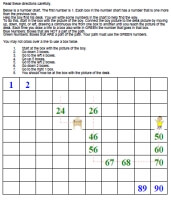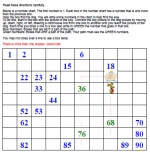Worksheets and No Prep Teaching Resources
Critical Thinking Puzzles
Make Puzzles

# Counting Chart Puzzles Puzzle Workbooks for Kids - Updated Each Month!

This page contains counting chart puzzles, which are puzzles that ask students to follow simple directions in order to unite a girl with her desk or a boy with his dog. By following directions like, "Move down three boxes," and "go to the left six boxes," students make their way to the desk or the dog. Easier puzzles have some of the path drawn, while more difficult puzzles are larger and have very little of the path filled in. These puzzles not only aid in developing critical thinking skills, but they are very satisfying as well, since there is an end goal to be achieved (getting to the desk or the dog).Counting Chart Puzzle: Easier - Follow the Directions (complete directions to the path are given)
Chart Always Begins with 1

Counting Chart Puzzle: Easier - Follow the Directions (complete directions to the path are given)
Chart can begin with any number (maximum number for any box is 100)

Counting Chart Puzzle: Follow the Directions (complete directions to the path are given)
The ending image is NOT shown - Student needs to circle the ending location themselves

Count by 2 to 12 Counting Chart Puzzle: Easier - Follow the Directions (complete directions to the path are given)
Easier: Number chart always begins with the count by number

Count by 2 to 12 Counting Chart Puzzle: Easier - Follow the Directions (complete directions to the path are given)
Large numbers; can start at any number

More Challenging - Counting Chart Puzzle: Easier - Find the Path and Follow DirectionsFollow the Directions Counting Chart Puzzle (half of path is given) Follow the Directions Counting Chart Puzzle (some of path is given) Follow the Directions Counting Chart Puzzle (little of path is given, but half of bad spots are given)

Hundreds Chart Pieces Puzzle

Following Directions

Hundreds Chart

Daily Critical Thinking Skills - Get Mixed Puzzles at Once!

Have a suggestion or would like to leave feedback?